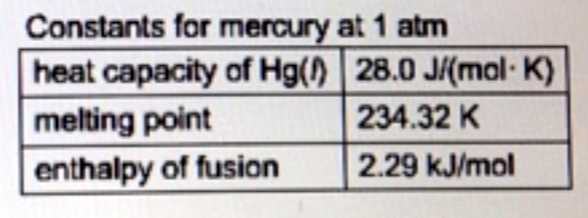# Problem: Calculate the heat energy (kJ) released when 23.6 g of liquid mercury at 25.00°C is converted to solid mercury at its melting point.

###### FREE Expert Solution
88% (367 ratings)
###### FREE Expert Solution

We’re being asked to calculate the amount of energy released when 23.6 g of mercury at 25.00 ˚C is converted to a solid at its melting point (234.32 K or –38.68 ˚C).

Note that cooling and freezing is an exothermic process, which means q is negative (–)

There are two heats involved in this problem:

1. q1 which is the heat in cooling 23.6 g of Hg from 25 ˚C to –38.68 ˚C

2. q2 which is the heat in freezing 23.6 g of Hg at –38.68 ˚C

We need to solve for each heat individually then add them together to get the final answer.

88% (367 ratings)###### Problem Details

Calculate the heat energy (kJ) released when 23.6 g of liquid mercury at 25.00°C is converted to solid mercury at its melting point.What scientific concept do you need to know in order to solve this problem?

Our tutors have indicated that to solve this problem you will need to apply the Heating and Cooling Curves concept. You can view video lessons to learn Heating and Cooling Curves. Or if you need more Heating and Cooling Curves practice, you can also practice Heating and Cooling Curves practice problems.

What is the difficulty of this problem?

Our tutors rated the difficulty ofCalculate the heat energy (kJ) released when 23.6 g of liqui...as high difficulty.

How long does this problem take to solve?

Our expert Chemistry tutor, Dasha took 10 minutes and 21 seconds to solve this problem. You can follow their steps in the video explanation above.

What professor is this problem relevant for?

Based on our data, we think this problem is relevant for Professor Crowell's class at UTA.